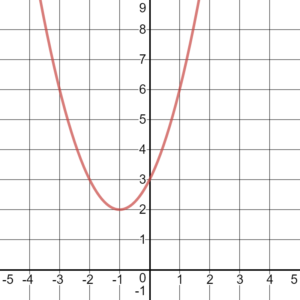## Complex Roots

### Learning Outcomes

• Find the complex roots of a quadratic function using the quadratic formula.
• Use the discriminant to determine whether a quadratic function has real or complex roots.

## Complex Roots

Now you will hopefully begin to understand why we introduced complex numbers at the beginning of this module. Consider the following function: $f(x)=x^2+2x+3$, and it’s graph below:Does this function have roots? It’s probably obvious that this function does not cross the $x$-axis, therefore it doesn’t have any $x$-intercepts. Recall that the $x$-intercepts of a function are found by setting the function equal to zero:

$x^2+2x+3=0$

In the next example we will solve this equation.  You will see that there are roots, but they are not $x$-intercepts because the function does not contain $(x,y)$ pairs that are on the $x$-axis. We call these complex roots.

By setting the function equal to zero and using the quadratic formula to solve, you will see that the roots are complex numbers.

### Example

Find the $x$-intercepts of the quadratic function. $f(x)=x^2+2x+3$

### Try It

The following video gives another example of how to use the quadratic formula to find complex solutions to a quadratic equation.

## The Discriminant

The quadratic formula not only generates the solutions to a quadratic equation, it tells us about the nature of the solutions. When we consider the discriminant, or the expression under the radical, ${b}^{2}-4ac$, it tells us whether the solutions are real numbers or complex numbers, and how many solutions of each type to expect. In turn, we can then determine whether a quadratic function has real or complex roots. The table below relates the value of the discriminant to the solutions of a quadratic equation.

Value of Discriminant Results
${b}^{2}-4ac=0$ One repeated rational solution
${b}^{2}-4ac>0$, perfect square Two rational solutions
${b}^{2}-4ac>0$, not a perfect square Two irrational solutions
${b}^{2}-4ac<0$ Two complex solutions

### A General Note: The Discriminant

For $a{x}^{2}+bx+c=0$, where $a$, $b$, and $c$ are real numbers, the discriminant is the expression under the radical in the quadratic formula: ${b}^{2}-4ac$. It tells us whether the solutions are real numbers or complex numbers and how many solutions of each type to expect.

### Example

Use the discriminant to find the nature of the solutions to the following quadratic equations:

1. ${x}^{2}+4x+4=0$
2. $8{x}^{2}+14x+3=0$
3. $3{x}^{2}-5x - 2=0$
4. $3{x}^{2}-10x+15=0$

We have seen that a quadratic equation may have two real solutions, one real solution, or two complex solutions.

Let’s summarize how the discriminant affects the evaluation of $\sqrt{{{b}^{2}}-4ac}$, and how it helps to determine the solution set.

• If $b^{2}-4ac>0$, then the number underneath the radical will be a positive value. You can always find the square root of a positive, so evaluating the Quadratic Formula will result in two real solutions (one by adding the square root, and one by subtracting it).
• If $b^{2}-4ac=0$, then you will be taking the square root of 0, which is 0. Since adding and subtracting 0 both give the same result, the “$\pm$” portion of the formula doesn’t matter. There will be one real repeated solution.
• If $b^{2}-4ac<0$, then the number underneath the radical will be a negative value. Since you cannot find the square root of a negative number using real numbers, there are no real solutions. However, you can use imaginary numbers. You will then have two complex solutions, one by adding the imaginary square root and one by subtracting it.

### Example

Use the discriminant to determine how many and what kind of solutions the quadratic equation $x^{2}-4x+10=0$ has.

## Contribute!

Did you have an idea for improving this content? We’d love your input.